• Shuffle
Toggle On
Toggle Off
• Alphabetize
Toggle On
Toggle Off
• Front First
Toggle On
Toggle Off
• Both Sides
Toggle On
Toggle Off
Toggle On
Toggle Off
Front

## Card Range To Study

throughPlay buttonPlay buttonProgress

1/29

Click to flip

Use LEFT and RIGHT arrow keys to navigate between flashcards;

Use UP and DOWN arrow keys to flip the card;

H to show hint;

 Quadrilateral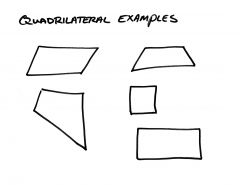A four-sided polygon. Parallelogram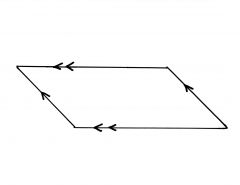A quadrilateral with both pairs of opposite sides parallel. If a quadrilateral is a parallelogram, then both pairs of opposite sides are...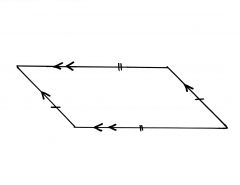congruent and parallel. If a quadrilateral is a parallelogram, then both pairs of opposite angles are...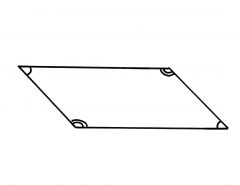congruent. If a quadrilateral is a parallelogram, then its diagonals...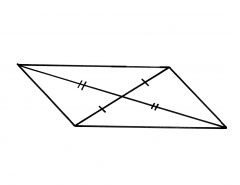bisect each other. If a quadrilateral is a parallelogram, then its consecutive angles are...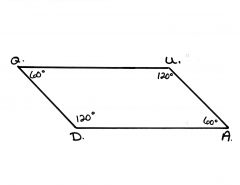supplementary. Parallelogram Opposite Sides Theorem: Opposite sides of a parallelogram are congruent. Parallelogram Opposite Angles Theorem: Opposite angles of a parallelogram are congruent. Parallelogram Consecutive Angles Theorem: Consecutive angles of a parallelogram are supplementary. Parallelogram Diagonals Theorem: The diagonals of a parallelogram bisect each other. Quadrilateral Parallel and Congruent Sides Theorem: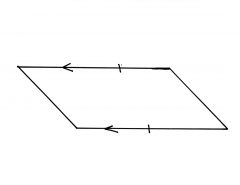If one pair of opposite sides of a quadrilateral is both parallel and congruent, then the quadrilateral is a parallelogram. Converse of the Quadrilateral Opposite Sides Theorem: If both pairs of opposite sides of a quadrilateral are congruent, then the quadrilateral is a parallelogram. Converse of the Quadrilateral Opposite Angles Theorem: If both pairs of opposite angles of a quadrilateral are congruent, then the quadrilateral is a parallelogram. Converse of the Quadrilateral Diagonals Theorem: If the diagonals of a quadrilateral bisect each other, then the quadrilateral is a parallelogram. Opposite, Parallel, Congruent Theorem: If one pair of opposite sides of a quadrilateral is both parallel and congruent, then the quadrilateral is a parallelogram. Rectangle Theorem: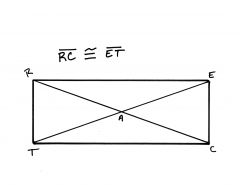If a parallelogram is a rectangle, then its diagonals are congruent. Converse of the Rectangle Theorem: If the diagonals of a parallelogram are congruent, then the parallelogram is a rectangle. If a quadrilateral is a rectangle, then opposite sides are ___________ and _____________. congruent and parallel If a quadrilateral is a rectangle, then opposite angles are ____________. congruent If a quadrilateral is a rectangle, then consecutive angles are ___________. supplementary If a quadrilateral is a rectangle, then diagonals are ______________ and ______________ each other congruent and bisect If a quadrilateral is a rectangle, then all four angles are ___________. right (90 degrees) Rhombus Diagonal Theorem: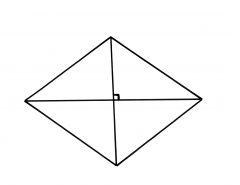The diagonals of a rhombus are perpendicular. Converse of the Rhombus Diagonal Theorem: If the diagonals of a parallelogram are perpendicular, then the parallelogram is a rhombus. Rhombus Angle Bisector Theorem: Each diagonal of a rhombus bisects a pair of opposite angles. If a quadrilateral is both a rhombus and a rectangle, it is a ____________. square Isosceles Trapezoid Base Angles Theorem: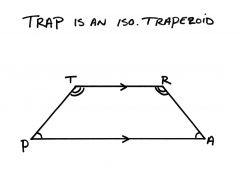Both pairs of base angles of an isosceles trapezoid are congruent. Isosceles Trapezoid Diagonal Theorem: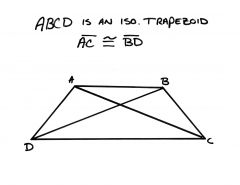The diagonals of an isosceles trapezoid are congruent. Trapezoid Median Theorem: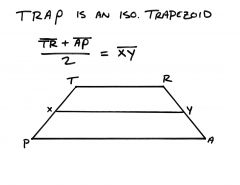The median of a trapezoid is parallel to the bases, and its measure is one-half the sum of the measures of the bases.